# String Text Extract

How to extract dollar amount from the following sample string?

There could be many instances of amounts as it is based on number of rows.

It is always formatted the same, dollar value, description, date.

410.44 USDChickFillA07/21/2022305.12 USDUPS07/21/2022

Hi @rjackson ,

Is this the expected output?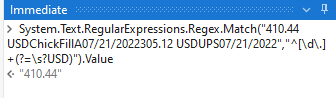If so then you can use a For Each Row in Data Table Activity and pass this into the Assign Activity:

``````System.Text.RegularExpressions.Regex.Match(CurrentRow(ColumnNameOrIndex).ToString,"^[\d\.]+(?=\s?USD)").Value
``````

ExtractDollarValue.xaml (8.3 KB)

Kind Regards,
Ashwin A.K

1 Like

please let us know if 305.12 USD is also to extract where the amount is glued to the year?
then a strategy as following could help: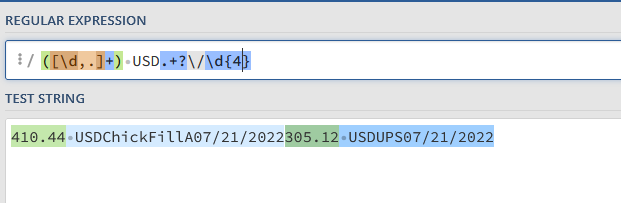by refering to group1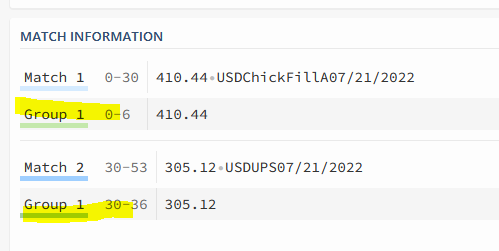`strPattern = ([\d,.]+) USD.+?\/\d{4}`
arrValues =

``````System.Text.RegularExpressions.Regex.Matches(YourString,strPattern).Cast(Of Match).Select(Function (x) x.Groups(1).Value).ToArray
``````

Welcome to UiPath community

``````System.Text.RegularExpressions.Regex.Match(YourString,"^[\d.]*(?=\s?USD)|(?<=\d{4})[\d.]*(?=\s?USD)").Value
``````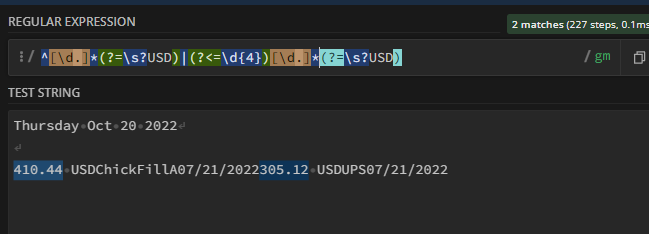Regards
Gokul

Hai @rjackson
Try this
To get 410.44

``````System.Text.RegularExpressions.Regex.Matches(YourString,"(?<=\d{2}\/\d{2}\/\d{4})[\d.]+(?=\sUSD)|^[\d.]+(?=\sUSD)")(0).ToString.Trim
``````

To get 305.12

``````System.Text.RegularExpressions.Regex.Matches(YourString,"(?<=\d{2}\/\d{2}\/\d{4})[\d.]+(?=\sUSD)|^[\d.]+(?=\sUSD)")(1).ToString.Trim
``````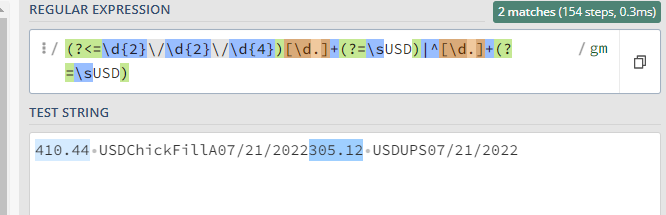This topic was automatically closed 3 days after the last reply. New replies are no longer allowed.